# dddtreecfs

Extract dual-tree/double-density wavelet coefficients or projections

## Syntax

``out = dddtreecfs(outputtype,wt,outputspec,outputindices)``
``out = dddtreecfs(outputtype,wt,outputspec,outputindices,'plot')``

## Description

example

````out = dddtreecfs(outputtype,wt,outputspec,outputindices)` extracts the coefficients or subspace projections from the 1-D or 2-D wavelet decomposition, `wt`. If `outputtype` equals `'e'`, `out` contains wavelet or scaling coefficients. If `outputtype` equals `'r'`, `out` contains wavelet or scaling subspace projections (reconstructions).```

example

````out = dddtreecfs(outputtype,wt,outputspec,outputindices,'plot')` plots the signal or image reconstruction or specified analysis coefficients. You can include the `'plot'` option anywhere after the `wt` input.```

## Examples

collapse all

Obtain the complex dual-tree wavelet transform of the 1-D noisy Doppler signal. Reconstruct an approximation based on the level-three detail coefficients in multiple ways.

Load the noisy Doppler signal. Obtain the complex dual-tree transform down to level 3.

```load noisdopp; wt = dddtree('cplxdt',noisdopp,3,'dtf1')```
```wt = struct with fields: type: 'cplxdt' level: 3 filters: [1x1 struct] cfs: {1x4 cell} ```

Plot a reconstruction of the original signal based on the level-three detail coefficients with `outputspec` set to `'scale'`.

`xr = dddtreecfs('r',wt,'scale',{3},'plot');`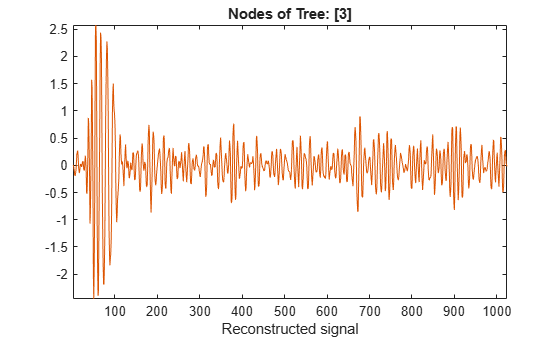The output `xr` is a 1-by-1 cell array. Generate the same reconstruction by using `'cumind'` and the level-three tree nodes. The first element of each vector in the cell array denotes the level, and the second element denotes the tree. Confirm the reconstructions are identical.

```outputindices = {[3 1];[3 2]}; xr2 = dddtreecfs('r',wt,'cumind',outputindices); max(abs(xr2-xr{1}))```
```ans = 0 ```

The output `xr2` is the same datatype as the original signal.

Load the noisy Doppler signal. Obtain the complex dual-tree transform down to level 3.

```load noisdopp; wt = dddtree('cplxdt',noisdopp,3,'dtf1')```
```wt = struct with fields: type: 'cplxdt' level: 3 filters: [1×1 struct] cfs: {[1×512×2 double] [1×256×2 double] [1×128×2 double] [1×128×2 double]} ```

Create a cell array of vectors to obtain the second- and third-level detail coefficients from each of the wavelet filter bank trees.

`outputindices = {[2 1]; [2 2]; [3 1]; [3 2]};`

The first element of each vector in the cell array denotes the level, or stage. The second element denotes the tree.

Extract the detail coefficients.

`detailCoeffs = dddtreecfs('e',wt,'ind',outputindices,'plot');`The output `detailCoeffs` is a 1-by-4 cell array. The cell array elements contain the wavelet coefficients corresponding to the elements in `outputindices`. For example, confirm `detailCoeffs{1}` contains the level-two detail coefficients from the first tree.

`max(abs(wt.cfs{2}(1,:,1)-detailCoeffs{1}))`
```ans = 0 ```

Load the noisy Doppler signal. Obtain the complex dual-tree transform down to level 3.

```load noisdopp; wt = dddtree('cplxdt',noisdopp,3,'dtf1');```

Create a cell array of vectors to obtain the second- and third-level detail coefficients from each of the wavelet filter bank trees.

`outputindices = {[2 1]; [2 2]; [3 1];[3 2]};`

The first element of each vector in the cell array denotes the level, or stage. The second element denotes the tree.

Create a structure array identical to the `wt` output of `dddtree` with all the coefficients equal to zero except the second- and third-level detail coefficients.

`out = dddtreecfs('e',wt,'cumind',outputindices,'plot');`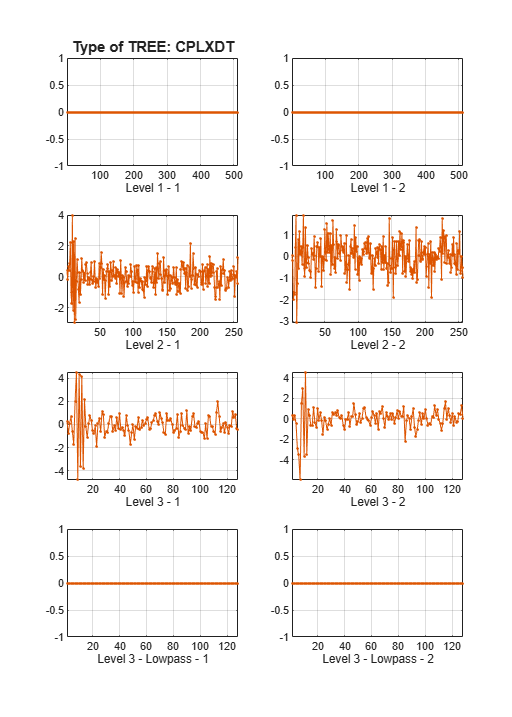Generate a reconstruction based on the second- and third-level detail coefficients.

`xr = idddtree(out);`

Generate two reconstructions, based on the second- and third-level detail coefficients. Confirm the sum of the two reconstructions is identical to `xr`.

```xr2 = dddtreecfs('r',wt,'scale',{2;3}); max(abs(xr-(xr2{1}+xr2{2})))```
```ans = 8.8818e-16 ```

Use the complex dual-tree wavelet transform to isolate diagonal features in an image at +45 and –45 degrees.

Load and display the `xbox` image.

```load xbox imagesc(xbox)```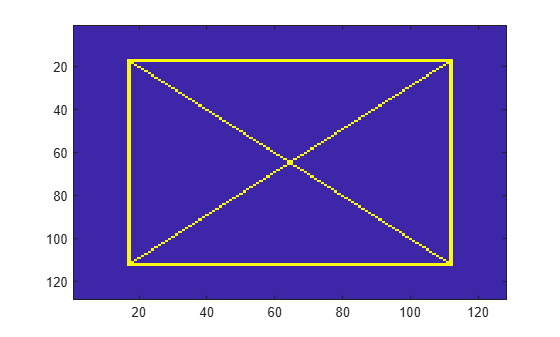Obtain the complex dual-tree wavelet transform down to level 3.

```fdf = dtfilters('FSfarras'); df = dtfilters('qshift10'); wt = dddtree2('cplxdt',xbox,3,fdf,df);```

Isolate the +45 and -45 diagonal image features in the level-one wavelet coefficients. Do this by creating a cell array of vectors specifying the tree nodes containing the diagonal details. The first element in the vector specifies the level. The three remaining elements specify the orientation, wavelet tree, and real and imaginary parts, respectively (see `dddtree2`).

```outputindices = {[1 3 1 1];[1 3 1 2];[1 3 2 1];[1 3 2 2]}; out = dddtreecfs('e',wt,'ind',outputindices,'plot');```This example shows how the analysis coefficients are distributed, depending on the transform, in the tree output of `dddtree` and `dddtree2`.

1-D Wavelet Transforms

Load in the noisy Doppler signal. Generate a four-level wavelet decomposition of the signal for each type of transform. Depending on the transform, different dimensions of the coefficient arrays correspond to orientation, wavelet tree, or real and imaginary parts.

Critically Sampled Discrete Wavelet Transform

```load noisdopp wt = dddtree('dwt',noisdopp,4,'sym4')```
```wt = struct with fields: type: 'dwt' level: 4 filters: [1x1 struct] cfs: {1x5 cell} ```

This is the usual nonredundant discrete wavelet transform. The first four elements of `wt.cfs` are the wavelet coefficients. The fifth element are the scaling coefficients.

Double-Density Wavelet Transform

`wt = dddtree('ddt',noisdopp,4,'filters1')`
```wt = struct with fields: type: 'ddt' level: 4 filters: [1x1 struct] cfs: {1x5 cell} ```

The third dimension of the 3-D wavelet coefficient arrays corresponds to the tree. The fifth element are the scaling coefficients.

Dual-Tree Complex Wavelet Transform

`wt = dddtree('cplxdt',noisdopp,4,'dtf1')`
```wt = struct with fields: type: 'cplxdt' level: 4 filters: [1x1 struct] cfs: {1x5 cell} ```

The third dimension of all the 3-D arrays in `cfs` corresponds to the real and imaginary parts. The first four elements of `cfs` are the wavelet coefficients, and `cfs{5}` are the scaling coefficients.

Reconstruct signals from the coefficients at the tree nodes `[1 1]`, `[5 2]`, `[3 1]`, and `[4 2]`. Plot the signals. The output is a cell array containing the reconstructions. The reconstructions are the same length as the original signal.

```outputindices = {[1 1];[5 2];[3 1];[4 2]}; XR = dddtreecfs('r',wt,'plot','ind',outputindices);```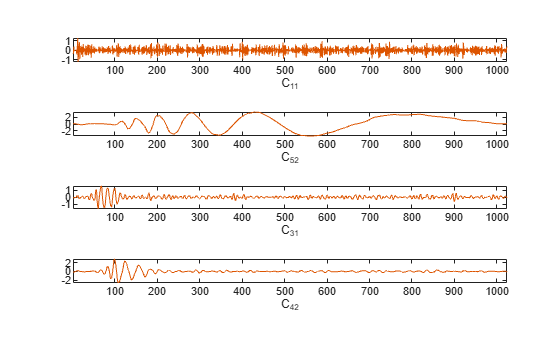Extract and plot the coefficients used to reconstruct the signals. The output is a cell array containing the coefficients of respective length: 512, 64, 128, and 64.

`XR = dddtreecfs('e',wt,'plot','ind',outputindices);`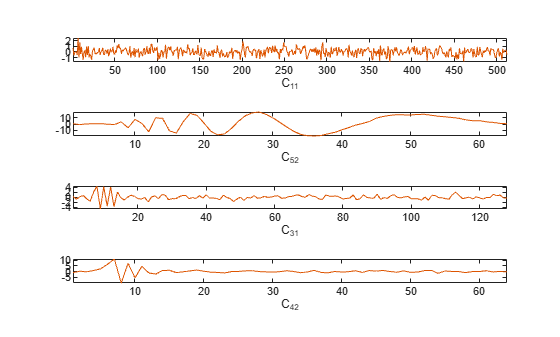Now use `'cumind'` instead of `'ind'`. The output `XR` is a signal of length 1024 in the first case, and a `'cplxdt'` dual-tree in the second one.

`XR = dddtreecfs('r',wt,'plot','cumind',outputindices);``XR = dddtreecfs('e',wt,'plot','cumind',outputindices);`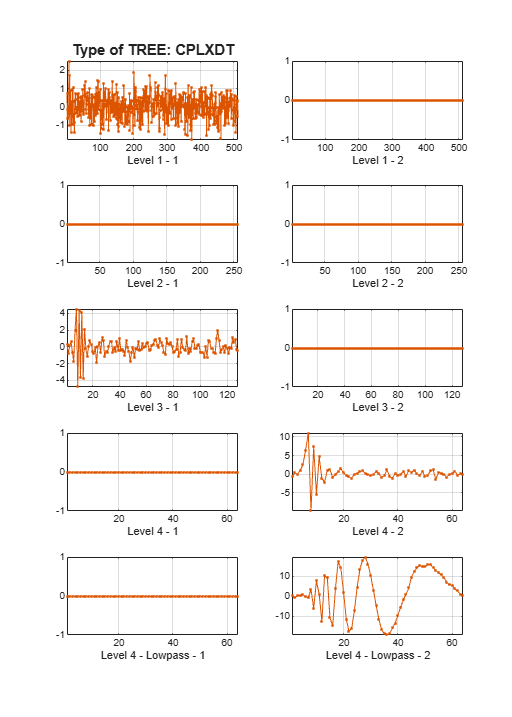Double-Density Dual-Tree Complex Wavelet Transform

`wt = dddtree('cplxdddt',noisdopp,4,'dddtf1')`
```wt = struct with fields: type: 'cplxdddt' level: 4 filters: [1x1 struct] cfs: {1x5 cell} ```

The third dimension of the 4-D wavelet coefficient arrays corresponds to the tree. The fourth dimension in the 4-D wavelet coefficient arrays and third dimension in the 3-D scaling coefficients array corresponds to the real and imaginary parts.

2-D Wavelet Transforms

Load in the 256-by-256 mask image. Generate a two-level wavelet decomposition of the image for each type of transform. Observe the dimensions of the output coefficients.

Critically Sampled Discrete Wavelet Transform

```load mask im = X; wt = dddtree2('dwt',im,3,'sym4')```
```wt = struct with fields: type: 'dwt' level: 3 filters: [1x1 struct] cfs: {1x4 cell} sizes: [10x2 double] ```

This is the usual nonredundant 2-D discrete wavelet transform. The third dimension in the 3-D wavelet coefficient arrays corresponds to the orientation. The scaling coefficients are the last element of `cfs`.

Real Oriented Dual-Tree Wavelet Transform

`wt = dddtree2('realdt',im,3,'dtf1')`
```wt = struct with fields: type: 'realdt' level: 3 filters: [1x1 struct] cfs: {1x4 cell} sizes: [11x2 double] ```

The fourth dimension in the 4-D wavelet coefficient arrays and third dimension in the 3-D scaling coefficients array correspond to the tree. The third dimension in the 4-D wavelet coefficient arrays corresponds to orientation.

Complex Oriented Dual-Tree Wavelet Transform

`wt = dddtree2('cplxdt',im,3,'dtf1')`
```wt = struct with fields: type: 'cplxdt' level: 3 filters: [1x1 struct] cfs: {[5-D double] [5-D double] [5-D double] [32x32x2x2 double]} sizes: [11x2 double] ```
`[size(wt.cfs{1});size(wt.cfs{2});size(wt.cfs{3})]`
```ans = 3×5 128 128 3 2 2 64 64 3 2 2 32 32 3 2 2 ```

The third dimension of the 5-D wavelet coefficient arrays represents the orientation. The fourth dimension in the 5-D arrays and third dimension in the 4-D scaling coefficients array represents the tree. The fifth dimension in the 5-D arrays and fourth dimension in the 4-D array represents the real and imaginary parts.

Double-Density Wavelet Transform

`wt = dddtree2('ddt',im,3,'filters1')`
```wt = struct with fields: type: 'ddt' level: 3 filters: [1x1 struct] cfs: {1x4 cell} sizes: [26x2 double] ```

The third dimension in the 3-D wavelet coefficient arrays represents the orientation.

Real Oriented Double-Density Wavelet Transform

`wt = dddtree2('realdddt',im,3,'self1')`
```wt = struct with fields: type: 'realdddt' level: 3 filters: [1x1 struct] cfs: {1x4 cell} sizes: [26x2 double] ```

The third dimension in the 4-D wavelet coefficient arrays represents the orientation. The fourth dimension in the 4-D arrays and third dimension in the 3-D scaling coefficients array represent the tree.

Complex Oriented Double-Density Wavelet Transform

`wt = dddtree2('cplxdddt',im,3,'self1')`
```wt = struct with fields: type: 'cplxdddt' level: 3 filters: [1x1 struct] cfs: {[5-D double] [5-D double] [5-D double] [32x32x2x2 double]} sizes: [26x2 double] ```
`[size(wt.cfs{1}) ; size(wt.cfs{2}) ; size(wt.cfs{3})]`
```ans = 3×5 128 128 8 2 2 64 64 8 2 2 32 32 8 2 2 ```

The third dimension of the 5-D wavelet coefficient arrays represents the orientation. The fourth dimension in the 5-D arrays and third dimension in the 4-D scaling coefficients array represents the tree. The fifth dimension in the 5-D arrays and fourth dimension in the 4-D array represents the real and imaginary parts.

Reconstruct and plot two images based on the second-level detail coefficients and scaling coefficients, respectively.

`XR = dddtreecfs('r',wt,'plot','scale',{2;4});`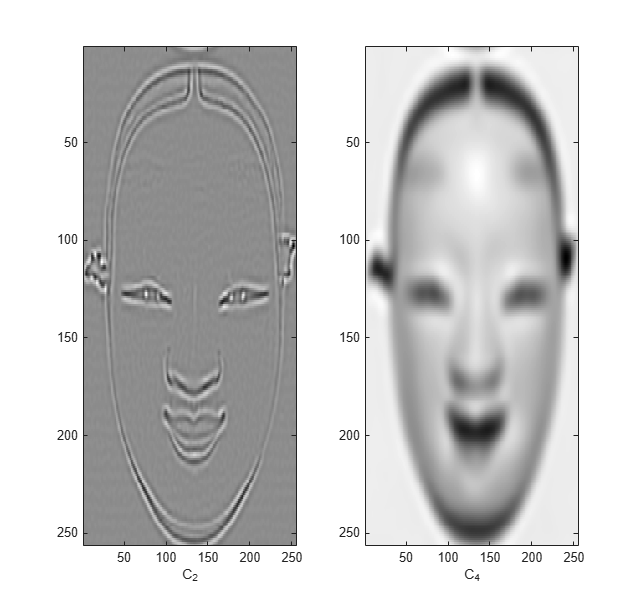The output `XR` is a cell array containing both 256-by-256 images.

Extract the coefficients used to produce the two images. The output is a cell array containing two dual-tree structures, one for each specified scale.

```XR = dddtreecfs('e',wt,'scale',{2;4}); XR{1}```
```ans = struct with fields: type: 'cplxdddt' level: 3 filters: [1x1 struct] cfs: {[5-D double] [5-D double] [5-D double] [32x32x2x2 double]} sizes: [26x2 double] ```
`XR{2}`
```ans = struct with fields: type: 'cplxdddt' level: 3 filters: [1x1 struct] cfs: {[5-D double] [5-D double] [5-D double] [32x32x2x2 double]} sizes: [26x2 double] ```

Confirm the only nonzero coefficients in each structure contained in `XR` are the level-two wavelet coefficients and scaling coefficients, respectively.

```dtInd = 1; [max(abs(XR{dtInd}.cfs{1}(:)));max(abs(XR{dtInd}.cfs{2}(:)));... max(abs(XR{dtInd}.cfs{3}(:)));max(abs(XR{dtInd}.cfs{4}(:)))]```
```ans = 4×1 0 143.9924 0 0 ```
```dtInd = 2; [max(abs(XR{dtInd}.cfs{1}(:)));max(abs(XR{dtInd}.cfs{2}(:)));... max(abs(XR{dtInd}.cfs{3}(:)));max(abs(XR{dtInd}.cfs{4}(:)))]```
```ans = 4×1 103 × 0 0 0 1.0545 ```

Use `'ind'` to reconstruct and display the four images based on the four lowpass components, respectively.

```outputindices = {[4 1 1];[4 2 1];[4 1 2];[4 2 2]}; XR = dddtreecfs('r',wt,'plot','ind',outputindices);```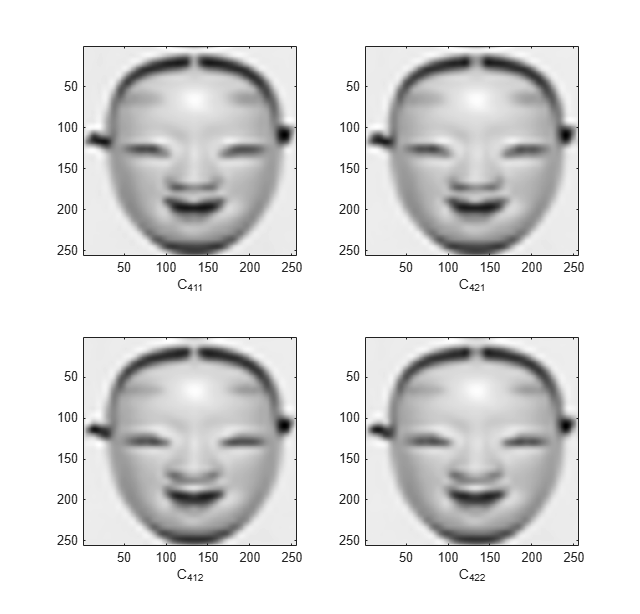The output `XR` is a cell array containing the four images. Each image is 256-by-256. Display the coefficients used to reconstruct the images.

`XR = dddtreecfs('e',wt,'plot','ind',outputindices);`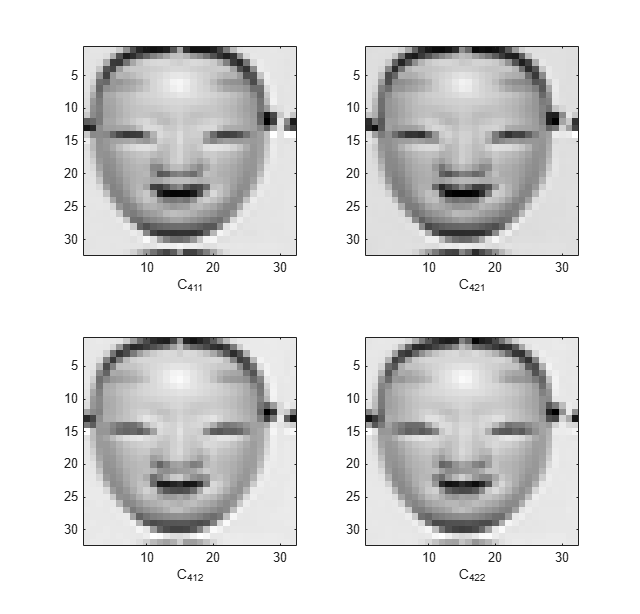The output `XR` is a cell array containing the four lowpass components. Each component is 32-by-32.

## Input Arguments

collapse all

Output type, specified as `'e'` or `'r'`. Use `'e'` to obtain the scaling or wavelet coefficients. Use `'r'` to obtain a projection, or reconstruction, onto the appropriate scaling or wavelet subspace.

Wavelet transform, specified as a structure. The structure array is the output of `dddtree` or `dddtree2`.

Output specification, specified as one of `'lowpass'`, `'scale'`, `'ind'`, or `'cumind'`. The output specifications are defined as follows:

• `'lowpass'` — Outputs the lowpass, or scaling, coefficients or a signal/image approximation based on the scaling coefficients. If you set the output specification to `'lowpass'`, do not specify `outputindices`. If the `outputtype` is `'e'`, `out` is a structure array with fields identical to the input structure array `wt` except that all wavelet (detail) coefficients are equal to zero. If the `outputtype` is `'r'`, `out` is a signal or image approximation based on the scaling coefficients. The signal or image approximation is equal in size to the original input to `dddtree` or `dddtree2`.

• `'scale'` — Outputs the coefficients or a signal/image approximation based on the scales specified in `outputindices`. If the `outputtype` is `'e'`, `out` is a cell array of structure arrays. The fields of the structure arrays in `out` are identical to the fields of the input structure array `wt`. The coefficients in the `cfs` field are all equal to zero except the coefficients corresponding to the scales in `outputindices`. If the `outputtype` is `'r'`, `out` is a signal or image approximation based on the scales in `outputindices`. The signal or image approximation is equal in size to the original input to `dddtree` or `dddtree2`.

• `'ind'` — Outputs the coefficients or a signal/image approximation based on the tree-position indices specified in `outputindices`. If the `outputtype` is `'e'`, `out` is a cell array of vectors or matrices containing the coefficients specified by the tree-position indices in `outputindices`. If the `outputtype` is `'r'`, `out` is a cell array of vectors or matrices containing signal or image approximations based on the corresponding tree-position indices in `outputindices`.

• `'cumind'` — Outputs the coefficients or a signal/image approximation based on the tree-position indices specified in `outputindices`. If the `outputtype` is `'e'`, `out` is a structure array. The fields of the structure array are identical to the fields of the input structure array `wt`. The coefficients in the `cfs` field are all equal to zero except the coefficients corresponding to the tree positions in `outputindices`. If the `outputtype` is `'r'`, `out` is a signal or image approximation based on the coefficients corresponding to the tree-position indices in `outputindices`.

Example: `'ind',{[1 1]; [1 2]}`

Output indices, specified as a cell array with scalar or vector elements. If `outputspec` equals `'scale'`, a scalar element selects the corresponding element in the `cfs` field of `wt`. If `outputspec` equals `'ind'` or `'cumind'`, the elements of `outputspec` are row vectors. The first element of the row vector corresponds to the element in the `cfs` field of `wt`. Subsequent elements in the row vector correspond to the indices of the array contained in the cell array element. For a description of the subsequent elements, see Distribution of Analysis Coefficients in Wavelet Tree Structure. For more information, see `dddtree` and `dddtree2`.

Example: `'scale',{1;2;3}`

## Output Arguments

collapse all

Signal or image reconstruction or coefficients, returned as a vector, matrix, structure array, cell array of vectors or matrices, or cell array of structure arrays. The form of `out` depends on the value of `outputspec` and `outputindices`.